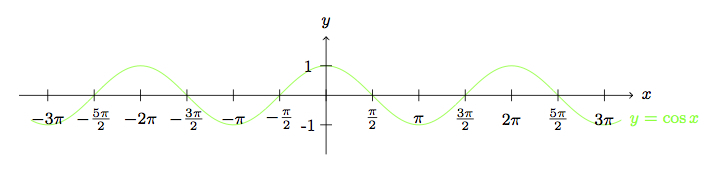# Trigonometric Identities and Rigid Motions

Alignments to Content Standards: G-CO.A.5 F-TF.A.2

1. Sketch graphs of $f(x) = \cos{x}$ and $g(x) = \sin{x}$.
2. Find a translation of the plane which maps the graph of $f$ to itself. Find a reflection of the plane which maps the graph of $f$ to itself. What trigonometric identities are associated with your translation and reflection?
3. Find a translation of the plane which maps the graph of $g$ to itself. Find a reflection of the plane which maps the graph of $f$ to itself. What trigonometric identities are associated with your translation and reflection?
4. Find a translation of the plane which maps the graph of $f$ to the graph of $g$. Find a reflection of the plane which maps the graph of $f$ to the graph of $g$. What trigonometric identities are associated with your translation and reflection?

## IM Commentary

The purpose of this task is to apply translations and reflections to the graphs of the functions $f(x) = \cos{x}$ and $g(x) = \sin{x}$ in order to derive some trigonometric identities. There are also rotations 180 degrees about points on the $x$-axis which preserve these graphs but these have the same impact as the reflections. For a slightly more challenging task, the teacher might request for all translations and/or reflections having the desired impact on the graphs.

The task illustrates F-TF.2 indirectly in the sense that in order to define and understand $\sin{x}$ and $\cos{x}$ for an arbitrary angle $x$, students must work with the unit circle. This aspect of the task is treated more fully in http://www.illustrativemathematics.org/content-standards/tasks/1704. If time permits, the two tasks can be done together. Otherwise, students may work with graphs of sine and cosine and argue informally, assuming that their ''apparent'' symmetries are genuine. Both approaches are important and, together, they show that the trigonometric identities investigated here are equivalent to symmetries of the graphs of sine and cosine.

## Solution

1. Here are the graphs of $f(x) = \cos{x}$ and $g(x) = \sin(x)$:The shapes of these graphs can be explained in terms of the definition of $\cos{x}$ and $\sin{x}$ for a real number $x$. If $(a,b)$ is the point on the unit circle obtained by rotating through $x$ radians from the point $(1,0)$ then $$(a,b) = (\cos{x},\sin{x}).$$ For example, if we rotate through $0$ radians this means we are still at $(1,0)$ and so $\cos{0} = 1$ and $\sin{0} = 0$. There are $2\pi$ radians in the circle so we come back to $(1,0)$ after rotating through $2\pi$ radians and this means that $\cos{(2\pi)} = 1$ and $\sin{(2\pi)} = 0$. This is the reason why the graphs of $\sin{x}$ and $\cos{x}$ are periodic: when $x$ changes by $2\pi$ (in the positive or negative direction), the values of $\sin{x}$ and $\cos{x}$ remain the same:

\begin{align} \cos{(x + 2\pi)} &= \cos{x}\\ \sin{(x + 2\pi)} &= \sin{x} \end{align}
2. In part (a) we explained the periodic behavior of the graphs in terms of the definitions of $\sin{x}$ and $\cos{x}$. We can see, looking at the graphs, that if we translate the graph of $f$ by any integer multiple of $2\pi$ we get the same graph. This means, expanding on the equations we found in part (a), for any integer $k$ we have $$\cos{(x \pm 2k\pi)} = \cos{x}.$$

Next we look for a reflection which maps the graph of $\cos{x}$ to itself. The $y$-axis is one example. Reflection about the $y$-axis sends $(x,\cos{x})$ to $(-x,\cos{x})$. The graph of $f$ contains the point $(-x,\cos{(-x)})$. Since the point $(-x,\cos{x})$ lies on the graph of $f$, this means that $$\cos{x} = \cos{(-x)}.$$

3. The analysis here is very similar to the solution to part (b). We can see, looking at the graphs, that if we translate the graph of $g$ by any integer multiple of $2\pi$ we get the same graph. This means, expanding on the equations we found in part (a), for any integer $k$ we have $$\sin{(x \pm 2k\pi)} = \sin{x}.$$

Next we look for a reflection which maps the graph of $\sin{x}$ to itself. The line $x = \frac{\pi}{2}$ is one example. Reflection about this line sends $(x,\sin{x})$ to $(\pi -x,\sin{x})$. The graph of $g$ contains the point $(\pi-x,\sin{(\pi-x)})$ so the fact that $(\pi-x,\sin{x})$ lies on the graph of $g$ means that $$\sin{x} = \sin{(\pi-x)}.$$

Alternatively, one could notice that the graph of $y=\sin(x)$ is preserved by rotation of 180 degrees around the origin. This expresses the fact that $\sin(x)$ is an odd function, and is algebraically encoded as $$\sin(-x)=-\sin(x)$$
4. Looking at the graphs, if we translate the graph of $f$ to the right by $\frac{\pi}{2}$ we get the graph of $g$. Symbolically, we have $f\left(x - \frac{\pi}{2}\right) = g(x)$. This means that $$\cos{\left(x-\frac{\pi}{2}\right)} = \sin{x}.$$

To find a reflection that maps the graph of $f$ to the graph of $g$, we can focus on the ''peaks'' of these graphs. The point $(0,1)$ lies on the graph of $f$ while the point $(\frac{\pi}{2},1)$ lies on the graph of $g$. To move $(0,1)$ to $(\frac{\pi}{2},1)$ we can reflect about $x = \frac{\pi}{4}$. We will find $f\left(\frac{\pi}{2} -x\right) = g(x)$ or $$\cos{\left(\frac{\pi}{2} -x\right)} = \sin{x}.$$

These last two identities look very similar and we can see how they are related by using identities that have been previously established. We have $\cos{(-v)} = \cos{v}$ for any real number $v$ and this relationship links the two identities above.